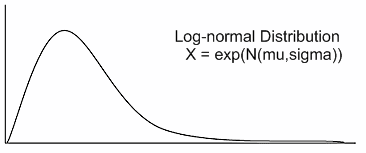# Volatility vs. LeverageMarch 1, 2009
722 Views

Recently I’ve been curious about volatility and leverage. Here’s the problem: let’s say you make or lose 10% of your total wealth based on the outcome of a coin flip. You make this bet a few times in a row. If you win then lose, you have 100*(1+10%) = \$110 then 110*(1-10%) = \$99. Likewise if you lose then win, 100*(1-10%) = \$90 then 90*(1+10%) = \$99. The more you play this game the more money you will lose, assuming the coin is fair. Basically you lose more when you’re up and win less when you’re down- both are bad.

This is one way of seeing why daily stock market returns must follow the log-normal distribution, which is right-skewed instead of a normal distribution. (in contrast to this Dutch book approach, you can also understand it as continuous returns are normally distributed, but daily/periodic are log-normal). If returns were symmetric, like the coin flipping game above, stocks would be pulled toward zero over time.And this would increase with volatility. For example, lever the coin flipping game 2x, so it’s 20% depending on if you win or lose (even without financing costs). 100*(1.2)*(.8) = \$96 = 100*(.8)*(1.2) so now you end up with even less. If the game is positive expec

Recently I’ve been curious about volatility and leverage. Here’s the problem: let’s say you make or lose 10% of your total wealth based on the outcome of a coin flip. You make this bet a few times in a row. If you win then lose, you have 100*(1+10%) = \$110 then 110*(1-10%) = \$99. Likewise if you lose then win, 100*(1-10%) = \$90 then 90*(1+10%) = \$99. The more you play this game the more money you will lose, assuming the coin is fair. Basically you lose more when you’re up and win less when you’re down- both are bad.

This is one way of seeing why daily stock market returns must follow the log-normal distribution, which is right-skewed instead of a normal distribution. (in contrast to this Dutch book approach, you can also understand it as continuous returns are normally distributed, but daily/periodic are log-normal). If returns were symmetric, like the coin flipping game above, stocks would be pulled toward zero over time.And this would increase with volatility. For example, lever the coin flipping game 2x, so it’s 20% depending on if you win or lose (even without financing costs). 100*(1.2)*(.8) = \$96 = 100*(.8)*(1.2) so now you end up with even less. If the game is positive expectation (such as +15%, -5%), i.e. you have an alpha generating strategy in market terminology, then leverage is a tradeoff between this downward pull of volatility and the upward pull of positive expected value.

It’s obvious that 0% leverage give \$0 downward pull, we showed 10\$ is \$1 down, 20% is \$4 down, a quick calc shows 100*1.3*.7 = \$91 i.e. \$9 down, etc. This simple plot illustrates the exponential trend of losses with respect to leverage/volatility:Fortunately the effect of levering up positive expectation is usually higher than the downward pull of volatility. The losses above are in terms of \$ but since the starting amount is 100, in terms of percents it would be the same. The game starts out at 10% volatility so 20% corresponds to 2:1 leverage, 30% 3:1, 10% 1:1 (no leverage), and 0% volatility means all cash/out of the market. If you have theoretical alpha of say 10%, 1:1 leverage will pull it down 1%. 2:1 leverage will pull it down 4%, but also double it to 20% for a net of +7% [=(20-10)-(4-1)]. 3:1 increases the volatility to 30% as above decreasing the return 9% but it raised it to 30% theoretical for a net of +5% [=(30-20)-(9-4)].

You can see in the example above that leverage helps but the benefit decreases (+7%, then +5%, then …). The alpha rises linearly with respect to leverage but returns are eaten away polynomially (degree 2) with respect to leverage on the bottom by volatility. Eventually the polynomial curve must cross the linear one and increasing leverage will then harm returns. The Kelly formula essentially finds this optimal crossover point. This is a toy example but perfectly applicable to trading.In the next note I will analyze the ultrashort ETF, SKF. It’s very interesting because it has to replicate the returns of the financial sector, leveraged 2x but inversely. So if the financial sector, XLF, goes up 3%, SKF has to go down 6% etc. The really intruiging thing to remember is that XLF’s returns must be right skewed, log-normal, or else it would steadily fall by te effect of volatility. However SKF is -1*XLF meaning that it is left skewed and should therefore be negative expectation, and furthermore suffer extra volatility problems because it is double leveraged. My question is how can an creation like SKF be possible in a no-arbitrage environment if it always loses money. And if it is possible, where does the money go that it’s losing… {can I have it?}